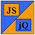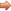# JavaScript & jQuery Tutorials

JS HomeJS ReferenceMath

## Math  static object

A static class containing mathematical constants and methods.

### Description

A suite of useful mathematical functions for use in our web pages.

• The `Math` global object is a static object which means we cannot instantiate objects of type `Math`.
• All the properties and methods of this class are also static and so we access these using `Math` followed by the name of the property or method we wish to use.

### Syntax

Signature Description
`Math.classPropertyName`Using the `Math` static object with a class property.
`Math.classMethodName()`Using the `Math` static object with a class method.

### Parameters

Parameter Description
`classPropertyName`The name of the class property we wish to use.
`classMethodName()`The name of the class method we wish to use.

### Class Properties

Class Property Description
`E`Euler's constant.
`LN2`Natural logarithm of 2.
`LN10`Natural logarithm of 10.
`LOG2E`Base 2 logarithm of E.
`LOG10E`Base 10 logarithm of E.
`PI`Circumference to diameter ratio of a circle.
`SQRT1_2`Square root of 1/2.
`SQRT2`Square root of 2.

### Instance Properties

We cannot instantiate `Math` objects and so there are no instance properties.

### Class Methods

Class Method Description
`abs()`Returns the absolute value of a number.
`acos()`Returns the arccosine of a number in radians.
`asin()`Returns the arcsine of a number in radians.
`atan()`Returns the arctangent quotient of two numbers.
`atan2()`Returns a numeric value between -pi and pi in radians.
`ceil()`Returns the smallest integer greater than or equal to a number.
`cos()`Returns the cosine of a number in radians.
`exp()`Returns the exponential value of a number.
`floor()`Returns the largest integer less than or equal to a number.
`log()`Returns the natural logarithm of a number.
`max()`Returns the largest of some numbers.
`min()`Returns the smallest of some numbers.
`pow()`Returns base to the exponent power.
`random()`Returns a random number between 0 and 1.
`round()`Returns the value of the number rounded to the nearest integer.
`sin()`Returns the sine of a number in radians.
`sqrt()`Returns the positive square root of a number.
`tan()`Returns the tangent of a number in radians.

### Instance Methods

We cannot instantiate `Math` objects and so there are no instance methods.

### Examples

``````
// Store properties in an array.
var mathProps = new Array(8);
mathProps = Math.E; // Euler's constant
mathProps = Math.LN2; // Natural log of 2
mathProps = Math.LN10; // Natural log of 10
mathProps = Math.LOG2E; // Base 2 log of E
mathProps = Math.LOG10E; // Base 10 log of E
mathProps = Math.PI; // Circumference to diameter ratio of a circle
mathProps = Math.SQRT1_2; // Square root of 1/2
mathProps = Math.SQRT2; // Square root of 2